# Determine the reactions and draw the shear and bending moment diagrams for the beams shown in Figs. P16.1–P16.5 by using the moment-distribution method. FIG. P16.1

#### Solutions

Chapter
Section
Chapter 16, Problem 1P
Textbook Problem

## Determine the reactions and draw the shear and bending moment diagrams for the beams shown in Figs. P16.1–P16.5 by using the moment-distribution method.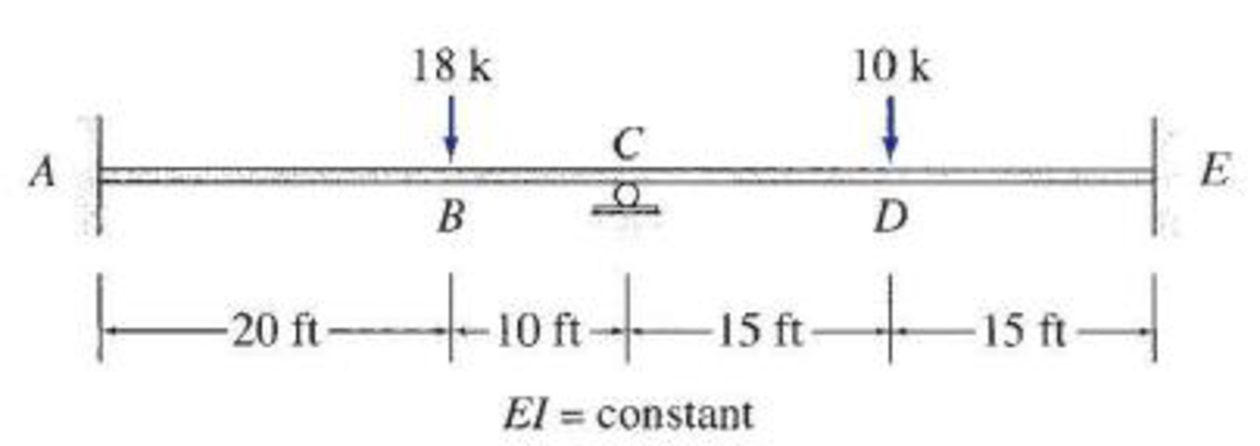FIG. P16.1

Expert Solution
To determine

Find the reaction and plot the shear and bending moment diagram.

### Explanation of Solution

Fixed end moment:

Formula to calculate the relative stiffness for fixed support IL and for roller support (34)(IL).

Formula to calculate the fixed moment for point load with equal length are PL8.

Formula to calculate the fixed moment for point load with equal length are PL8.

Formula to calculate the fixed moment for point load with unequal length are Pab2L2 and Pa2bL2.

Formula to calculate the fixed moment for UDL is WL212.

Calculation:

Consider the flexural rigidity EI of the beam is constant.

Show the free body diagram of the entire beam as in Figure 1.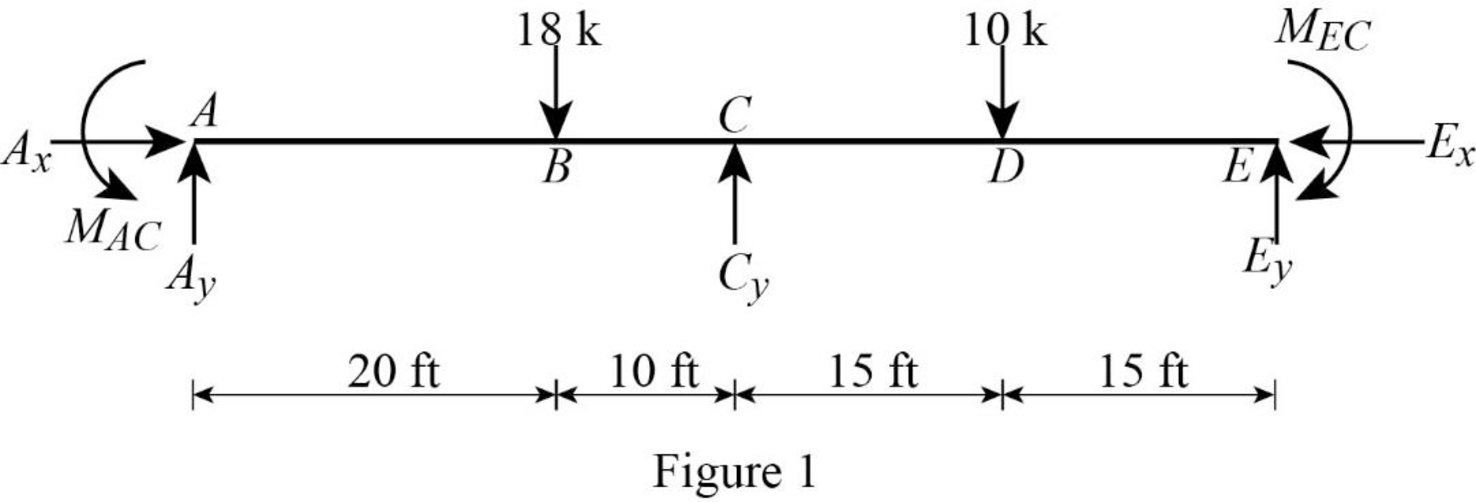Refer Figure 1,

Calculate the relative stiffness KCA for part CA of the beam:

KCA=I20ft+10ft=I30

Calculate the relative stiffness KCE for part CE of the beam:

KCE=I15ft+15ft=I30

In the above beam, only joint C is free to rotate. Hence, calculate the distribution factor at joint C.

Calculate the distribution factor DFCA for member AC of the beam.

DFCA=KCAKCA+KCE

Substitute I30 for KCA and I30 for KCE.

DFCA=I30I30+I30=0.5

Calculate the distribution factor DFCA for part CE of the beam.

DFCE=KCEKCA+KCE

Substitute I30 for KCA and I30 for KCE.

DFCE=I30I30+I30=0.5

Check for sum of distribution factor:

DFCE+DFCA=1

Substitute 0.5 for DFCE and 0.5 for DFCA.

0.5+0.5=1

Hence, OK.

Calculate the fixed end moment for AC.

FEMAC=18×20×102(30)2=40kft

Calculate the fixed end moment for CA.

FEMCA=18×202×10(30)2=80kft

Calculate the fixed end moment for CE.

FEMCE=10×308=37.5kft

Calculate the fixed end moment for EC.

FEMEC=10×308=37.5kft

Show the calculation of final moments using moment distribution method as in Table 1.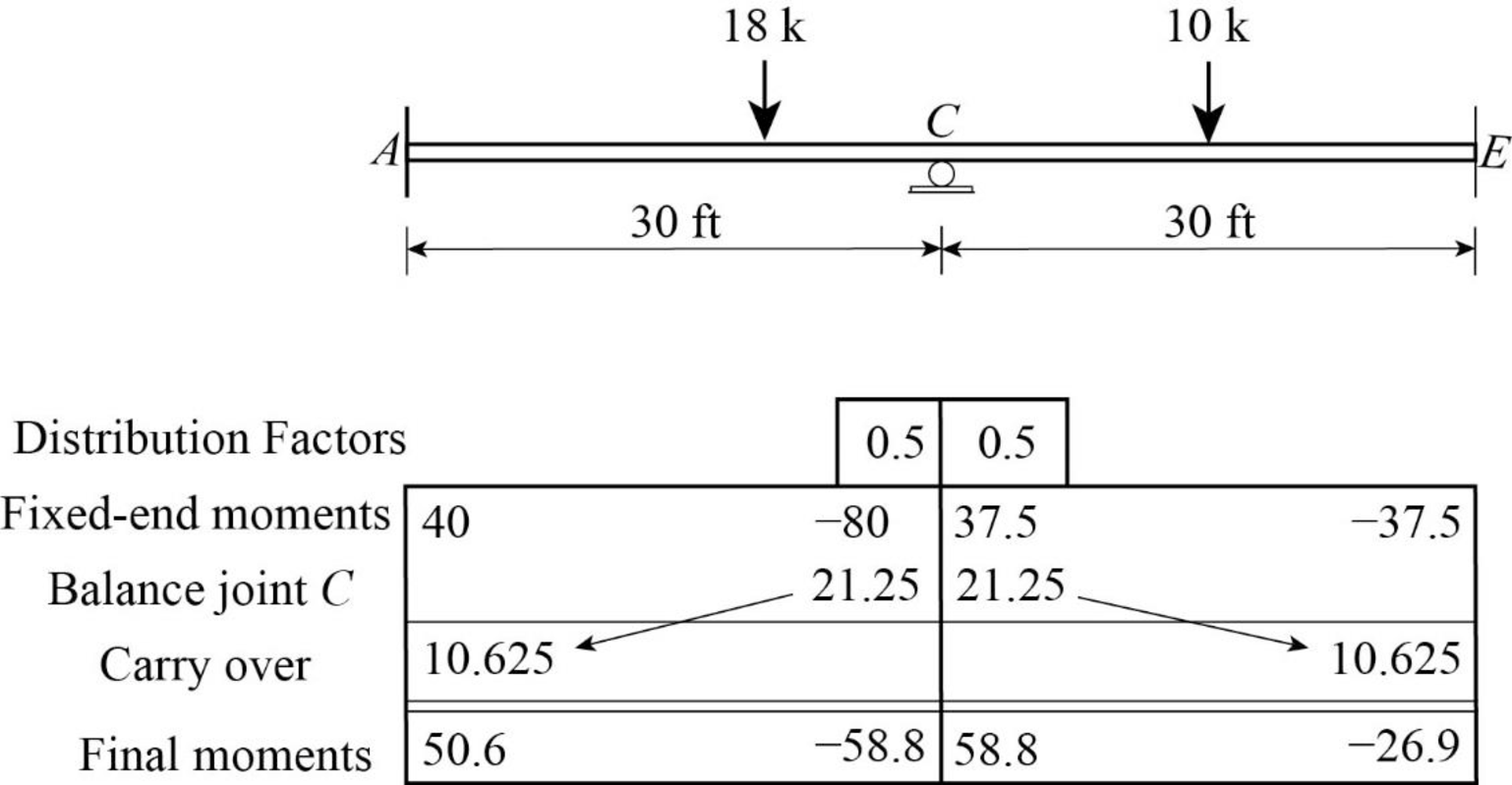Consider the member AC of the beam:

Show the free body diagram of the member AC as in Figure 2.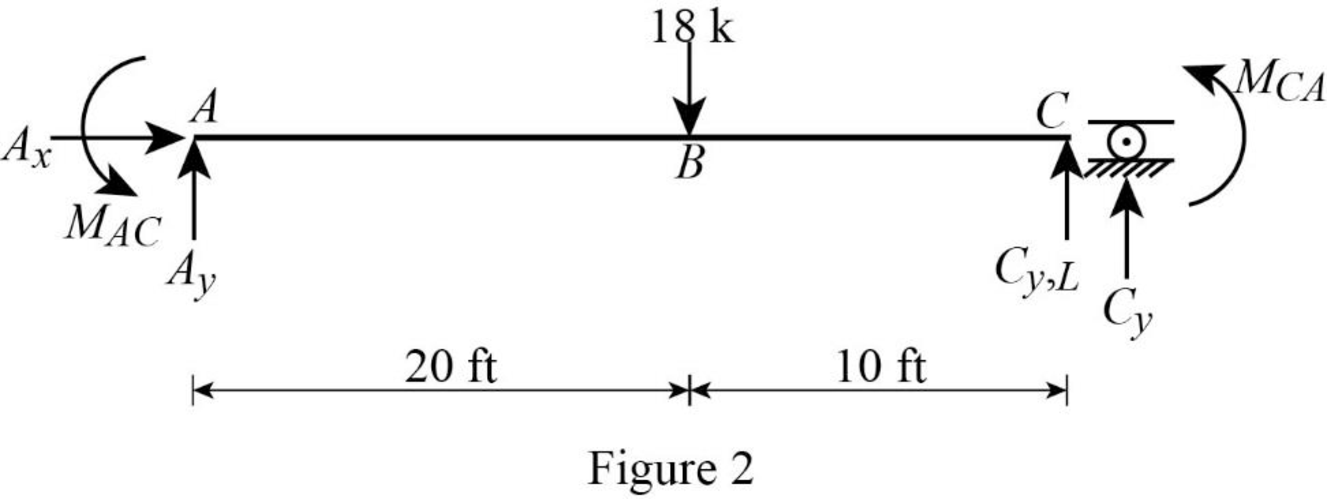Calculate the vertical reaction at the left end of the joint C by taking moment about point A.

+MA=0Cy,L(30)18×(20)+50.62558.75=0Cy,L(30)=368.125Cy,L=368.12530Cy,L=12.271k

Calculate the horizontal reaction at point A by resolving the horizontal equilibrium.

+Fx=0Ax=0

Calculate the vertical reaction at point A by resolving the vertical equilibrium.

+Fy=0Ay18+Cy,L=0Ay18+12.271=0Ay=5.729k

Consider the member CE of the beam:

Show the free body diagram of the member CE as in Figure 3.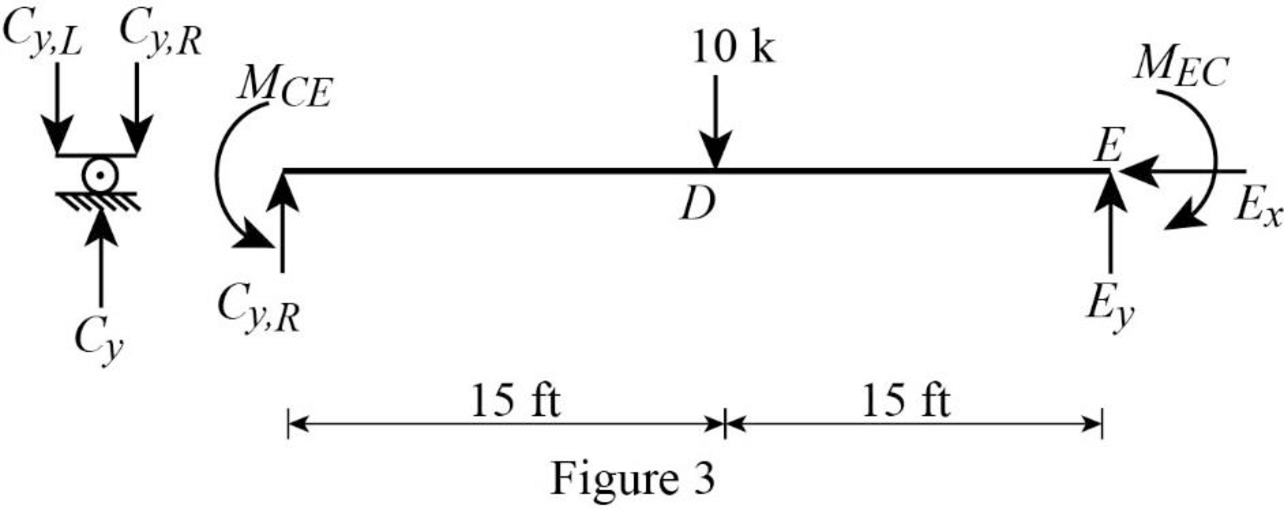Calculate the vertical reaction at the right end of the joint C by taking moment about point E.

+ME=0Cy,R(30)+10×(15)26.875+58.75=0Cy,R(30)=181.875Cy,R=181.87530Cy,R=6.063k

Calculate the horizontal reaction at point E by resolving the horizontal equilibrium.

+Fx=0Ex=0

Calculate the vertical reaction at point E by resolving the vertical equilibrium.

+Fy=0Ey10+Cy,R=0Ey10+6.063=0Ey=3.937k

Calculate the total reaction at point C.

Cy=Cy,R+Cy,L

Substitute 6.063k for Cy,R and 12.271k for Cy,L.

Cy=6.063+12.271=18.334k

Show the reaction of the beam in Figure 4.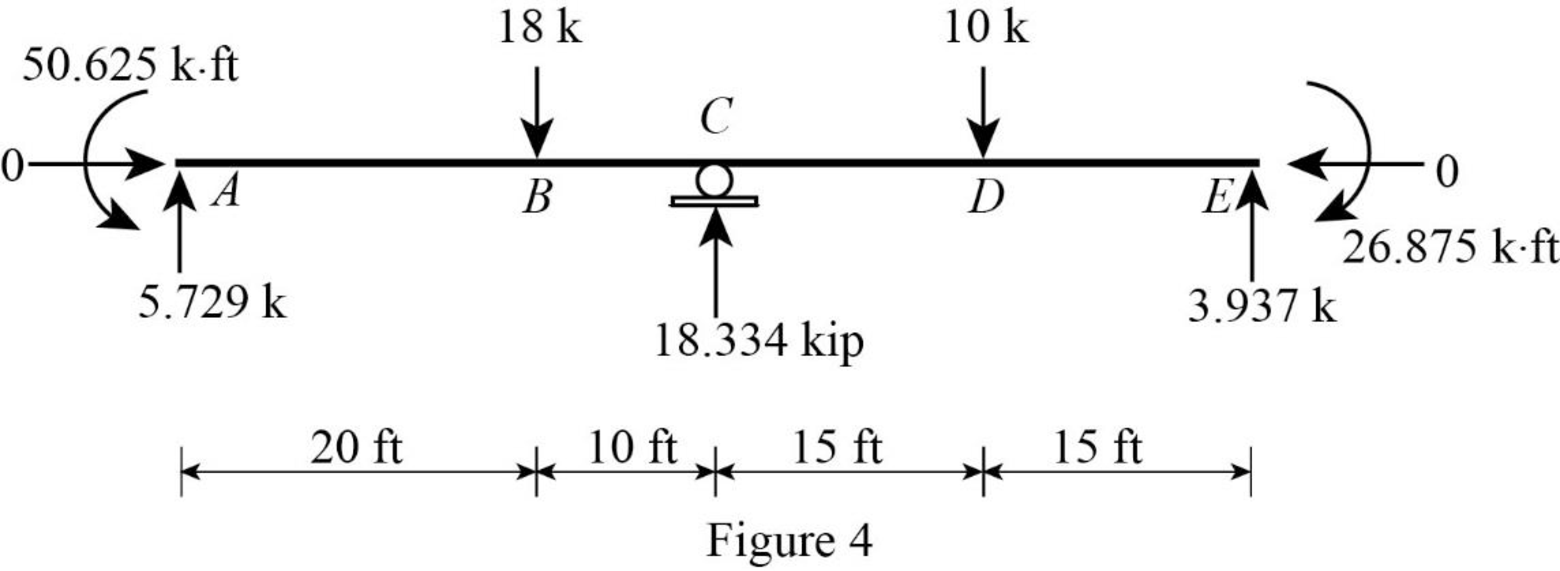Refer Figure 4,

Shear diagram:

Point A:

SA,L=0SA,R=5.729k

Point B:

SB=5.72918=12.271k

Point C:

SC,L=12.271kSC,R=12.271+18.334=6.063k

Point D:

SD,L=6.063kSD,R=6.06310=3.937k

Point E:

SE,L=3.937kSE,R=3.937k+3.937=0k

Plot the shear force diagram of the beam as in Figure 5.Refer Figure 4,

Bending moment diagram:

Point A:

MA=50.625kft

Point B:

MB=50.625+(5.729×20)=63.955kft

Point C:

MC=58.75kft58.8kft

Point D:

MD=58.8+(6.063×15)=32.145k

Point E:

ME=26.875kft26.9kft

Plot the bending moment diagram of the beam as in Figure 6.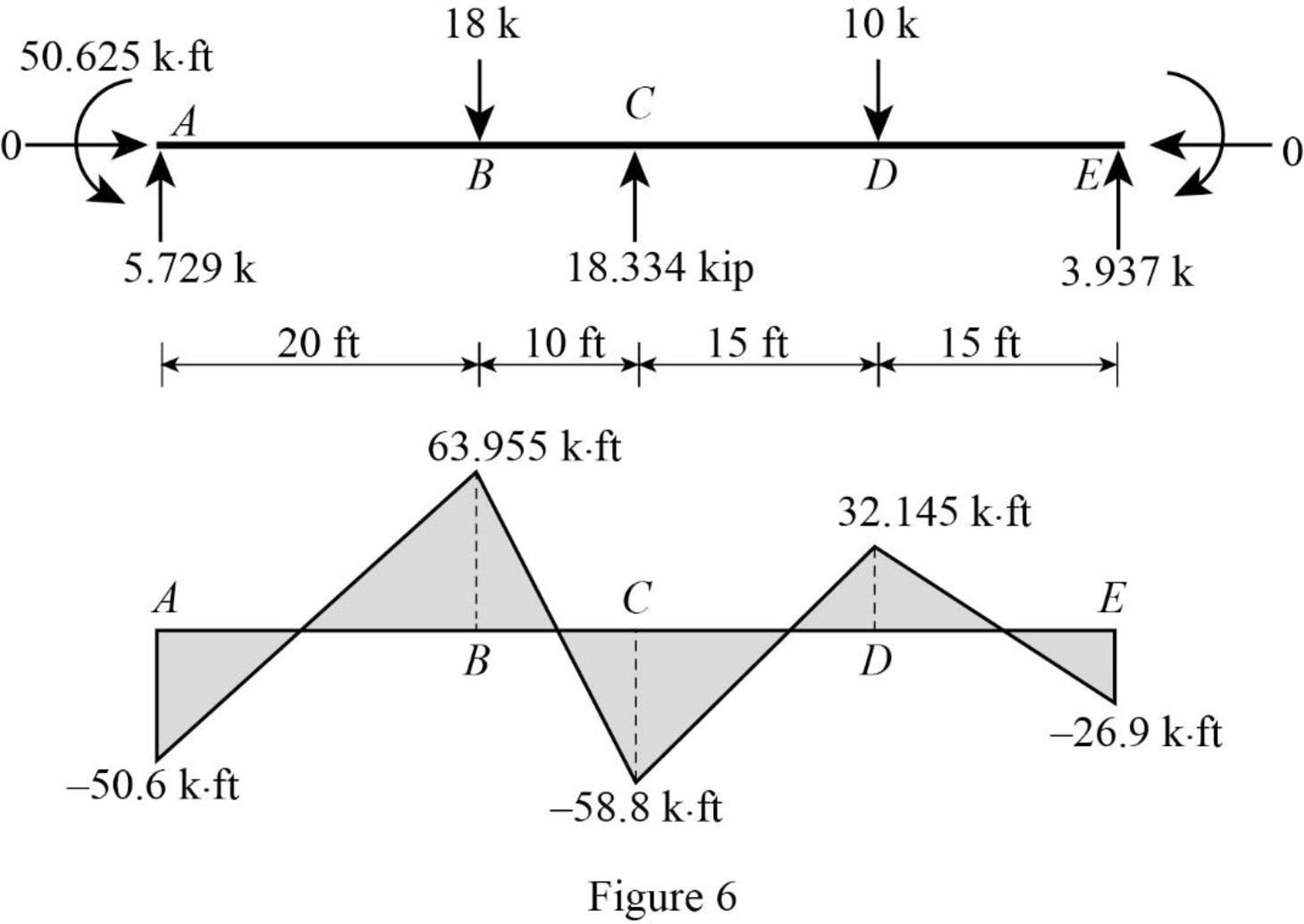### Want to see more full solutions like this?

Subscribe now to access step-by-step solutions to millions of textbook problems written by subject matter experts!

Get Solutions

### Want to see more full solutions like this?

Subscribe now to access step-by-step solutions to millions of textbook problems written by subject matter experts!

Get Solutions

Find more solutions based on key concepts
Show solutions
Imagine the water level in the tank described in Problem 9.38 rises at a rate of 0.1 in/s. Knowing the diameter...

Engineering Fundamentals: An Introduction to Engineering (MindTap Course List)

How might the diffusion of innovation theory be applied to this project?

Principles of Information Systems (MindTap Course List)

What e some of the benefits and shortcomings of using CASE tools for process modeling activities?

Systems Analysis and Design (Shelly Cashman Series) (MindTap Course List)

What causes condensed water to leak from the air conditioning system?

Automotive Technology: A Systems Approach (MindTap Course List)

What is risk management?

Management Of Information Security

Which port must be open for RDP traffic to cross a firewall?

Network+ Guide to Networks (MindTap Course List)

What is manufacturing?

Precision Machining Technology (MindTap Course List)

Search Skills Selecting Search Terms Search text that you send to a search engine, such as Google, Bing, or Yah...

Enhanced Discovering Computers 2017 (Shelly Cashman Series) (MindTap Course List)

If your motherboard supports ECC DDR3 memory, can you substitute non-ECC DDR3 memory?

A+ Guide to Hardware (Standalone Book) (MindTap Course List)

What should be done with a leaking cylinder if the leak cannot be stopped?

Welding: Principles and Applications (MindTap Course List)